﻿ Theoretical Analysis of Surface Characteristics of Aspheric Lenses - Hyperion Optics

# Theoretical Analysis of Surface Characteristics of Aspheric Lenses

### Abstract:

Aspheric lenses are becoming more and more important in some high-precision optical systems. And, like other optical lenses, the surface must meet the same quality standards in terms of surface shape accuracy and surface roughness. In this paper, are aspheric less studied deeply from the theory of surface characteristics, the calculation method of surface features of aspheric lenses, aspheric lenses surface characteristics, and calculation formula are given, and the characteristics of surface fitting error of aspheric lenses are analyzed. Taking K9 glass aspheric optical lens, for example, the surface characteristic function is discussed in detail. The results show that the surface characteristic function of aspheric lenses has higher precision, and its fitting error is better than + 30nm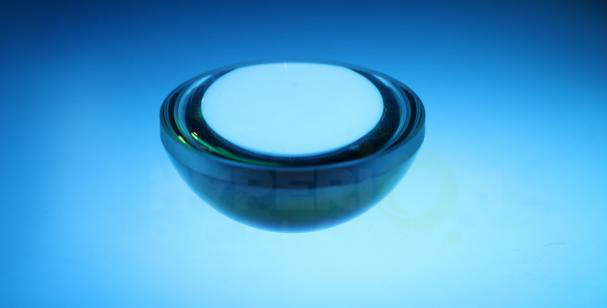### Keyword: aspheric surface, surface characteristic, fitting error

As an important optical element, aspheric lenses occupies an important position in the optical system. Aspheric lenses function in 3-4 times than spherical lenses in the optical system. Mainly because of spherical aberration of aspherical lenses in the optical system, such as lateral deviation, axis deviation and angle deviation, which limits the application of spherical lens in the optical system. The aspheric lens has the ability to eliminate spherical aberration, thus greatly improving the sphere of use of aspheric lenses in the optical system.

The research of aspheric lenses is mainly in two aspects. 1 using the optical design software (such as CODE V and ZEMAX) design of aspheric lenses, so as to solve the problem of light propagation and imaging in the aspheric lens; the research mainly focuses on the production process of an aspheric surface.

In foreign countries, the surface fitting of the aspheric surface has been studied deeply, and the fitting method of the aspheric surface is less. Hector proposed a curve algorithm suitable for conic aspheric; based on linear least squares method, ZHANG proposed aspheric parameters calculation algorithm; By changing the conic constant values of K and using the max-min value of the least squares method, G ugsa gets the surface contour standard. The above method considers only the surface of a single standard surface. When measuring the aspheric surface in the day, the estimation of the cone constant K will be biased due to the different coefficients of the polynomial. In this paper, the surface characteristic function of the aspheric surface is established by theoretical analysis. The surface characteristic function of the aspheric surface is discussed by taking K9 glass as an example, and the fitting error is analyzed.

Related Content
• ## Round Concave Mirriors

March 29, 2019Round concave mirrors are catoptric imaging. Mirrors( including convex) are reflecting back for imaging rather than get through the light, ray of light follows the law of reflection, that is what we c...view
• ## Classification of Optical Lenses and Their Functions

February 2, 2021Optical glass refers to glass that can change the direction of light propagation and can change the relative spectral distribution of ultraviolet, visible or infrared light. Optical lenses are made of...view
•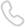Call us on:
+86-25-83307137
•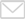Email us:
rfq@hypoptics.com
•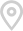DanYang Industrial Zone Danjie Road #100, Danyang,China
• Sitemap
• DanYang Industrial Zone Danjie Road #100, Danyang,China
• +86-25-83307137
• +86-25-86626312
• rfq@hypoptics.com
•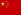• Hyperion Optics USA Inc.
1981 RT 27 STE 204
EDISON, NJ 08817
• +1 (908) 899-1918
• rfq-us@hypoptics.com
•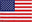•